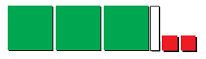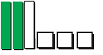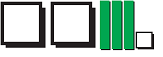# Characteristics of polynomials#### All in One Place

Everything you need for better grades in university, high school and elementary.#### Learn with Ease

Made in Canada with help for all provincial curriculums, so you can study in confidence.#### Instant and Unlimited Help

0/2
##### Intros
###### Lessons
1. What is a polynomial?
• Review on Variables, Coefficients, and Expressions
• What are Monomials, Binomials, and Trinomials?
• What are the Degree, Leading Term, and Constant term of a polynomial?
• Name polynomials based on degree: Quadratic, Cubic, Quartic, Quintic, etc.
2. How to find the degree of a polynomial?
0/13
##### Examples
###### Lessons
1. Determine the number of terms and the name for each of the expression.
1. $- 5{y^2}$
2. $2{x^2} + 4x + 6$
3. $- 7.3$
4. $y^3 + ay + nx - x^2$
2. Find the degree and number of terms for each of the following expression.
1. $2xy - 3{y^2} + 6$
2. $4{a^3} + a$
3. $- 4$
3. Write the expression for each set of the algebra tiles.

1.2.3.4. Peter wants to buy some comic books and magazines. A bookstore sells comic books for $12 each and magazines for$8 each.
1. Write an expression to represent the money Peter needs to spend on both comic books and magazines.
2. How much does Peter need to spend if he wants 9 comic books and 3 magazines?
3. Write a new expression for Peter's expenditure on books if the bookstore raises the price of a comic book to $15 and a magazine to$10.
0%
##### Practice
###### Topic Notes
A polynomial consists of constants, variables and exponents. Polynomials are named based on the number of terms they have. Polynomials with 1 term are called monomials. Those with 2 terms are called binomials, and the ones with 3 terms are called trinomials.